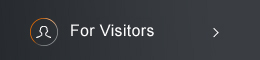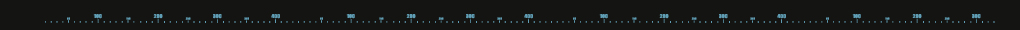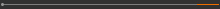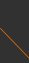Sponsored by[ Events ]

﻿
Activity Search
Sort out
 select all Seminars and Talks Conference and Workshop Lectures and Courses ElseField
 select all Number Theory and Representation Theory Algebraic Geometry Differential Geometry and Geometric Analysis Differential Equations and Stochastic Analysis Scientific Computing Interdisciplinary Studies Laboratory of Data Science Harmonic Analysis OthersYear
 select 2015 2016 2017 2018 2019 2020 2021 2022 2023 2024NCTS Nonlinear PDE and Analysis Seminar14:30 - 15:30, October 27, 2021 (Wednesday) Cisco Webex, Online seminar (線上演講 Cisco Webex) On Strong Convergence of a Modified Elliptic Regularization to Advection Equations (II) Daisuke Kawagoe (Kyoto University) Abstract: We consider an advection equation with the homogeneous inflow boundary condition in a bounded domain with the piecewise $C^1$ boundary. When we apply the standard elliptic regularization to this boundary value problem, its convergence rate as the viscosity parameter $\epsilon$ tends to $0$ is of order $\epsilon^{1/4}$ in some cases and not known in general. In this talk, we show that a family of solutions obtained by a modified elliptic regularization converges to the solution to the original problem with order $\epsilon^{1/2}$ under the assumption that the original solution has $H^1$ regularity. We also derive better convergence rates with more assumptions on domains, vector fields, and regularity of the original solution.   JOIN WEBEX MEETING(C) 2021 National Center for Theoretical Sciences